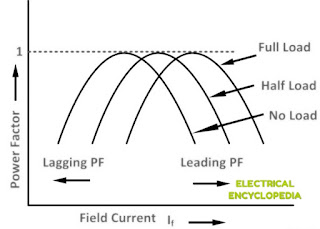# V Curve of a Synchronous Motor

0
560

## V Curve of a Synchronous Motor

#### In this article we will study about the V Curves of Synchronous Motor.Graph plotted between “Armature Current flowing through Stator” and “field current” at a constant load is known as V curve of synchronous motor.V Curves of Synchronous Motor for no load, half load and full load is shown in the figure below.Fig-1: Family of V curves of synchronous motor

### INVERTED V CURVES

#### A family of curves can be obtained by plotting the power factor versus field current at a constant load of synchronous motor. These curves are called Inverted V Curves.Fig-2: Inverted Curve